Courses

# Sampling, JIT, TQM & Graphical Method - MCQ Test 2

## 25 Questions MCQ Test RRB JE for Mechanical Engineering | Sampling, JIT, TQM & Graphical Method - MCQ Test 2

Description
This mock test of Sampling, JIT, TQM & Graphical Method - MCQ Test 2 for Mechanical Engineering helps you for every Mechanical Engineering entrance exam. This contains 25 Multiple Choice Questions for Mechanical Engineering Sampling, JIT, TQM & Graphical Method - MCQ Test 2 (mcq) to study with solutions a complete question bank. The solved questions answers in this Sampling, JIT, TQM & Graphical Method - MCQ Test 2 quiz give you a good mix of easy questions and tough questions. Mechanical Engineering students definitely take this Sampling, JIT, TQM & Graphical Method - MCQ Test 2 exercise for a better result in the exam. You can find other Sampling, JIT, TQM & Graphical Method - MCQ Test 2 extra questions, long questions & short questions for Mechanical Engineering on EduRev as well by searching above.
QUESTION: 1

Solution:
QUESTION: 2

### Consider the following statements: Linear programming model can be applied to: 1. Line balancing problem 2. Transportation problem 3. Project ma nagement Of these statements:

Solution:

Linear programming model can be applied to line balancing problem and transportation problem but not to project management.

QUESTION: 3

### Assertion (A): Sampling plans with acceptance number greater than zero are generally better than sampling plans with acceptance number equal to zero. Reason (R): Sampling plans with acceptance number greater than zero have a larger sample size as compared to similar sampling plans with acceptance number equal to zero.

Solution:
QUESTION: 4

The primal of a LP problem is maximization of objective function with 6 variables and 2 constraints.

Which of the following correspond to the dual of the problem stated?
1. It has 2 variables and 6 constraints
2. It has 6 variables and 2 constraints
3. Maximization of objective function
4. Minimization of objective function

Select the correct answer using the codes given below:

Solution:
QUESTION: 5

Which one of the following is not the characteristic of acceptance sampling?

Solution:
QUESTION: 6

Solution to Z =  4x1 + 6x2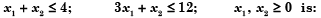Solution: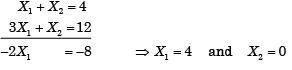QUESTION: 7

Assertion (A): In attribute control of quality by sampling, the sample size has to be larger than variable control.

Reason (R): Variables are generally continuous, and attributes have few discrete levels.

Solution:
QUESTION: 8

In a linear programming problem, which one of the following is correct for graphical method?

Solution:
QUESTION: 9

Assertion (A): In dodge romig sampling tables, the screening inspection of rejected lots is also included.

Reason (R): Dodge romig plans are indexed at an LTPD of 10%

Solution:
QUESTION: 10

Which of the following are correct in respect of graphically solved linear programming problems?

1. The region of feasible solution has concavity property.
2. The boundaries of the region are lines or planes.
3. There are corners or extreme points on the boundary

Select the correct answer using the code given below:

Solution:
QUESTION: 11

Consider the following statements in respect of double sampling plan:

1. Average number of pieces inspected is double that of single sampling
2. Average number of pieces inspected is less than that for single sampling
3. Decision to accept or reject the lot is taken only after the inspection of both samples
4. Decision to accept or reject the lot is reached sometimes after one sample and sometimes after two samples

Which of these statements are correct?

Solution:
QUESTION: 12

In case of solution of linear programming problem using graphical method, if the constraint line of one of the non-redundant constraints is parallel to the objective function line, then it indicates

Solution:

All points on the line is a solution. So there are infinite no of optimal solutions.

QUESTION: 13

The product is assembled from parts A and B. The probability of defective parts A and B are 0.1 and 0.2 respectively. Then the probability of the assembly of A and B to be non defective is:

Solution:

It is a case of mutually independent events. Probability that the part A is non-defective = 1 - 0.1 = 0.9

Probability that Part-B is non-defective, = 1 - 0.2 = 0.8

Hence, probability that both Part-A and Part-B are non-defective = 0.9 x 0.8 = 0.72.

QUESTION: 14

Which one of the following statements is NOT correct?

Solution:
QUESTION: 15

In a study to estimate the idle time of a machine, out of 100 random observations the machine was found idle on 40 observations. The total random observations required for 95% confidence level and ± 5% accuracy is:

Solution: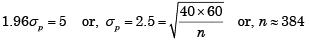QUESTION: 16

A variable which has no physical meaning, but is used to obtain an initial basic feasible solution to the linear programming problem is referred to as:

Solution:
QUESTION: 17

A control chart is established with limits of ± 2 standard errors for use in monitoring samples of size n = 20. Assume the process to be in control. What is the likelihood of a sample mean falling outside the control limits?

Solution:
QUESTION: 18

Consider the following statements regarding the characteristics of the standard form of a linear programming problem:

1. All the constraints are expressed in the form of equations.
2. The right-hand side of each constraint equation is non-negative.
3. All the decision variables are non-negative.

Which of these statements are correct?

Solution:
QUESTION: 19

The management is interested to know the percentage of idle time of equipment. The trial study showed that percentage of idle time would be 20%. The number of random observations necessary for 95% level of confidence and ± 5% accuracy is:

Solution: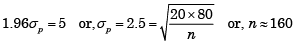QUESTION: 20

A variable which has no physical meaning, but is used to obtain an initial basic feasible solution to the linear programming problem is referred to as:

Solution:
QUESTION: 21

For a confidence level of 95% and accuracy ± 5%, the number of cycles to be timed in a time study is equal to: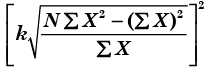Where, N = Number of observations taken; X = X1, X2, ...., XN are individual observations. What is the value of K?

Solution:

For 95% confidence level
K = 2
S = Accuracy = 0.05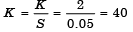QUESTION: 22

If m is  the number of constraints in a linear programming with two variables x and y and non-negativity constraints x ≥ 0, y ≥ 0; the feasible region in the graphical solution will be surrounded by how many lines?

Solution:

Constraints = 3 the feasible region is surrounded by more two lines x-axis and y-axis.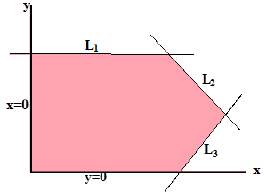QUESTION: 23

If one state occurred four times in hundred observations while using the work-sampling technique, then the precision of the study using a 95% confidence level will be:

Solution: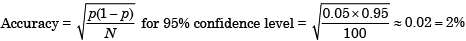QUESTION: 24

Which of the following conditions are necessary of applying linear programming?

1. There must be a well defined objective function
2. The decision variables should be interrelated and non-negative
3. The resources must be in limited supply

Solution:
QUESTION: 25

Match List-I with List-II and select the correct answer: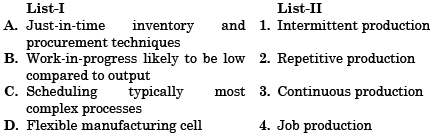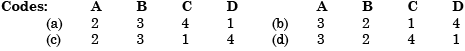Solution: